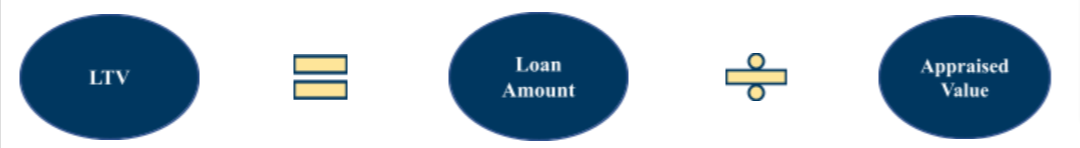# Loan-to-Value Ratio (LTV) Definition

The Loan-to-Value (LTV) ratio is a metric that helps lenders understand the amount of risk they will be taking on when lending to a borrower.## What is LTV?

The Loan-to-Value (LTV) ratio is a metric that helps lenders understand the amount of risk they will be taking on when lending to a borrower.

## How is it useful?

The ratio answers vital questions for lenders such as:

• What is the minimum down payment?
• What is the interest rate?
• How much risk does this deal hold?
• What is the loan amount?
• How much skin will the borrower have in the deal?
• How much skin will the lender have in the deal?

## How do lenders calculate the LTV?LTV = 62.50%
LTV = \$250,000 / \$400,000

Appraised Values = \$400,000
Property 1 = \$95,000
Property 2 = \$105,000
Property 3 = \$80,000
Property 4 = \$120,000

Amount Borrowed = \$250,000

## What does a 62.50% LTV mean?

The lender will lend 62.50% of the funds needed to acquire or refinance the properties. The borrower will need to put up the remaining 37.50% of the capital.

## What is the optimal LTV ratio?

Lenders strive for an LTV ratio between 60% to 75% which means borrowers will need to invest between 25% to 40% of the capital required.

## Low DSCR vs High LTV

Loans are identified as low risk when they have a low LTV ratio as the lender will have less equity in the project. The greatest benefit of a low LTV ratio is that the borrower will have access to the lowest interest rates available. Low LTV ratios decrease the interest rate, decrease the deal’s risk, increase the borrower’s down payment and decrease the loan amount.
Loans are identified as high risk when they have a high LTV ratio as the borrower will have less equity in the project. These loans will require a higher interest rate as the lender is acquiring more risk. Furthermore, lenders may require mortgage insurance for the loan as a safety net. High LTV ratios increase the interest rate, increase the deal’s risk, decrease the borrower down payment and increase the loan amount.

## Apply for a quick estimate now

Lender / Broker? Request a demo